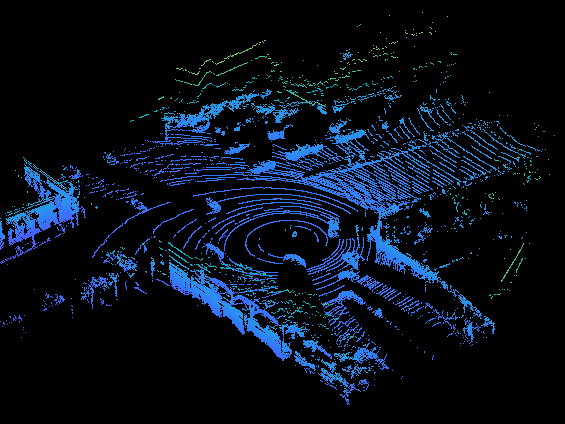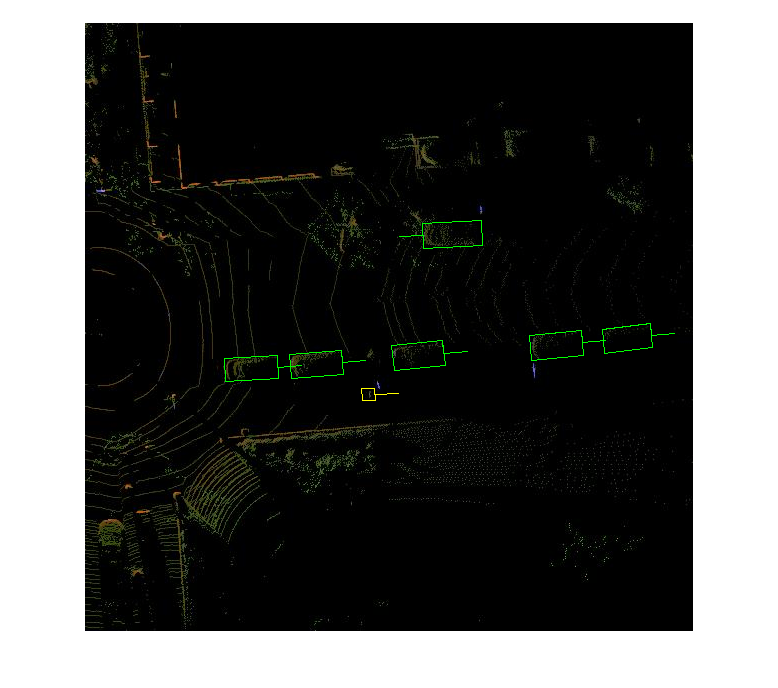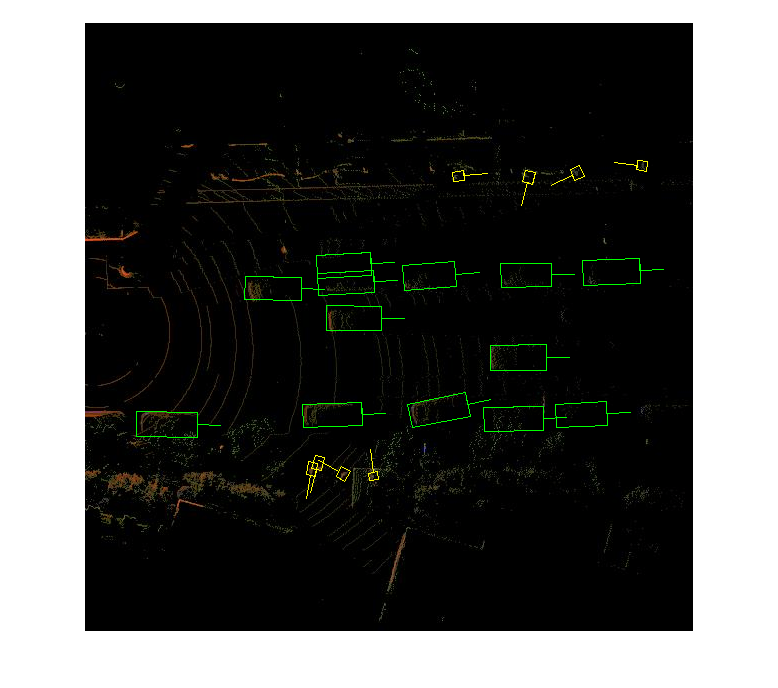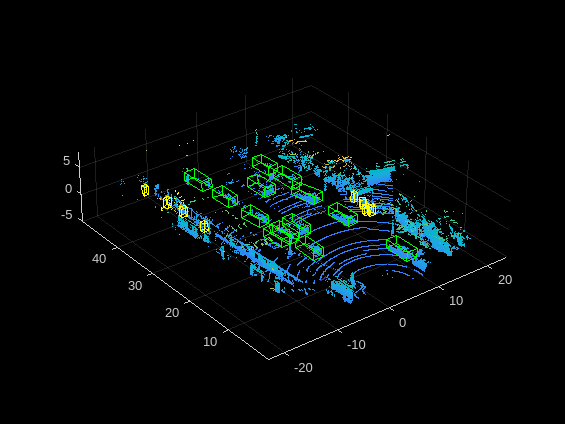# Lidar Object Detection Using Complex-YOLO v4 Network

This example shows how to train a Complex-YOLO v4 network to perform object detection on point clouds.

The Complex-YOLO  approach is effective for lidar object detection as it directly operates on bird's-eye-view RGB maps that are transformed from the point clouds. In this example, using the Complex-YOLO approach, you train a YOLO v4  network to predict both 2-D box positions and orientation in the bird's-eye-view frame. You then project the 2-D positions along with the orientation predictions back onto the point cloud to generate 3-D bounding boxes around the object of interest.

This example uses a subset of the PandaSet data set  that contains 2560 preprocessed organized point clouds. Each point cloud covers 360 degrees of view and is specified as a 64-by-1856 matrix. The point clouds are stored in PCD format and their corresponding ground truth data is stored in the `PandaSetLidarGroundTruth.mat` file. The file contains 3-D bounding box information for three classes, which are car, truck, and pedestrian. The size of the data set is 5.2 GB.

Download the PandaSet data set from the given URL using the `helperDownloadPandasetData` helper function, defined at the end of this example.

```outputFolder = fullfile(tempdir,'Pandaset'); lidarURL = ['https://ssd.mathworks.com/supportfiles/lidar/data/' ... 'Pandaset_LidarData.tar.gz']; helperDownloadPandasetData(outputFolder,lidarURL);```

Depending on your internet connection, the download process can take some time. The code suspends MATLAB® execution until the download process is complete. Alternatively, you can download the data set to your local disk using your web browser and extract the file. If you do so, change the `outputFolder` variable in the code to the location of the downloaded file. The download file contains `Lidar`, `Cuboids`, and `semanticLabels` folders, which contain the point clouds, cuboid label information, and semantic label information respectively.

This example implements two variants of the complex YOLO v4 object detectors:

• `complex-yolov4-pandaset` — Standard complex YOLO v4 network trained on bird's-eye-view generated from point clouds of the PandaSet data set

• `tiny-complex-yolov4-pandaset` — Lightweight complex YOLO v4 network trained on bird's-eye-view images generated from point clouds of the PandaSet data set

The pretrained networks are trained on three object categories: car, truck and pedestrian.

```modelName = 'tiny-complex-yolov4-pandaset'; mdl = downloadPretrainedComplexYOLOv4(modelName); net = mdl.net; ```

Create a file datastore to load the PCD files from the specified path using the `pcread` function.

```path = fullfile(outputFolder,'Lidar'); lidarData = fileDatastore(path,'ReadFcn',@(x) pcread(x));```

Load the 3-D bounding box labels of the car, truck, and pedestrian objects.

```gtPath = fullfile(outputFolder,'Cuboids','PandaSetLidarGroundTruth.mat'); data = load(gtPath,'lidarGtLabels'); Labels = timetable2table(data.lidarGtLabels); boxLabels = Labels(:,2:end);```

Display the full-view point cloud.

```figure ptCld = read(lidarData); ax = pcshow(ptCld.Location); set(ax,'XLim',[-50 50],'YLim',[-40 40]); zoom(ax,2.5); axis off;```### Create Bird's-eye-view Image from Point Cloud Data

The PandaSet data consists of full-view point clouds. For this example, crop the full-view point clouds and convert them to a bird's-eye-view images using the standard parameters. These parameters determine the size of the input passed to the network. Selecting a smaller range of point clouds along the x-, y-, and z-axes helps you detect objects that are closer to the origin.

```xMin = -25.0; xMax = 25.0; yMin = 0.0; yMax = 50.0; zMin = -7.0; zMax = 15.0; ```

Define the dimensions for the bird's-eye-view image.

```bevHeight = 608; bevWidth = 608;```

Find the grid resolution.

```gridW = (yMax - yMin)/bevWidth; gridH = (xMax - xMin)/bevHeight;```

Define the grid parameters.

`gridParams = {{xMin,xMax,yMin,yMax,zMin,zMax},{bevWidth,bevHeight},{gridW,gridH}};`

Convert the training data to bird's-eye-view images by using the `transformPCtoBEV` helper function, attached to this example as a supporting file. You can set `writeFiles` to `false` if your training data is already present in the `outputFolder`.

```writeFiles = true; if writeFiles transformPCtoBEV(lidarData,boxLabels,gridParams,outputFolder); end```

### Create Datastore Objects for Training

```dataPath = fullfile(outputFolder,'BEVImages'); imds = imageDatastore(dataPath);```

```labelPath = fullfile(outputFolder,'Cuboids','BEVGroundTruthLabels.mat'); load(labelPath,'processedLabels'); blds = boxLabelDatastore(processedLabels);```

Remove the data that has no labels from the training data.

`[imds,blds] = removeEmptyData(imds,blds);`

Split the data set into a training set for training the network and a test set for evaluating the network. Use 60% of the data for training set and the rest for testing..

```rng(0); shuffledIndices = randperm(size(imds.Files,1)); idx = floor(0.6 * length(shuffledIndices));```

Split the image datastore into training and test sets.

```imdsTrain = subset(imds,shuffledIndices(1:idx)); imdsTest = subset(imds,shuffledIndices(idx+1:end));```

Split the box label datastore into training and test sets.

```bldsTrain = subset(blds,shuffledIndices(1:idx)); bldsTest = subset(blds,shuffledIndices(idx+1:end));```

Combine the image and box label datastores.

```trainData = combine(imdsTrain,bldsTrain); testData = combine(imdsTest,bldsTest);```

Use the `validateInputDataComplexYOLOv4` helper function, attached to this example as a supporting file, to detect:

• Samples with an invalid image format or that contain NaNs

• Bounding boxes containing zeros, NaNs, Infs, or are empty

• Missing or noncategorical labels.

The values of the bounding boxes must be finite and positive and cannot be NaNs. They must also be within the image boundary with a positive height and width.

```validateInputDataComplexYOLOv4(trainData); validateInputDataComplexYOLOv4(testData);```

### Preprocess Training Data

Preprocess the training data to prepare for training. The `preprocessData` helper function, listed at the end of the example, applies the following operations to the input data.

• Resize the images to the network input size.

• Scale the image pixels in the range [0 1].

• Set `isRotRect` to `true` to return the rotated rectangles.

```networkInputSize = [608 608 3]; isRotRect = true; preprocessedTrainingData = transform(trainData,@(data)preprocessData(data,networkInputSize,isRotRect));```

`data = read(preprocessedTrainingData);`

Display an image with the bounding boxes.

```I = data{1,1}; bbox = data{1,2}; labels = data{1,3}; helperDisplayBoxes(I,bbox,labels);```Reset the datastore.

`reset(preprocessedTrainingData);`

### Modify Pretrained Complex-YOLO V4 Network

The Complex-YOLO V4 network uses anchor boxes estimated from the training data to have better initial estimate corresponding to the type of data set and to help the network learn to predict the boxes accurately.

First, because the training images vary in size, use the `transform` function to preprocess the training data and resize all the images to the same size.

Specify the number of anchors:

• `complex-yolov4-pandaset model` — Specify 9 anchors

• `tiny-complex-yolov4-pandaset model` — Specify 6 anchors

Use the `estimateAnchorBoxes` function to estimate the anchor boxes. For reproducibility, set the random seed. Then pass the estimated anchor boxes to the `configureComplexYOLOV4` function to arrange them in the correct order for the training. Set `isRotRect` to false because the rotation angle is not necessary for the bounding boxes to estimate the anchors.

```rng(0) isRotRect = false; trainingDataForEstimation = transform(trainData,@(data)preprocessData(data,networkInputSize,isRotRect)); numAnchors = 6; [anchorBoxes,meanIoU] = estimateAnchorBoxes(trainingDataForEstimation,numAnchors)```
```anchorBoxes = 6×2 23 52 11 13 12 17 19 8 25 60 10 10 ```
```meanIoU = 0.7925 ```

Specify the class names to use for training.

```classNames = {'Car' 'Truck' 'Pedestrain'};```

Configure the pretrained model for training using the `configureComplexYOLOV4` function. This function returns a the modified layer graph, network output names, reordered anchor boxes, and anchor box masks to select anchor boxes to use in the detected heads. Configure the detection head of the YOLO v4 model to predict the angle regression along with bounding boxes, the objectness score, and classification scores.

`[net,networkOutputs,anchorBoxes] = configureComplexYOLOv4(net,classNames,anchorBoxes,modelName);`

### Specify Training Options

Specify these training options.

• Set the number of epochs to 90.

• Set the mini batch size to 8. Stable training is possible with higher learning rates when higher mini batch size is used. Set this value depending on the memory available.

• Set the learning rate to 0.001.

• Set the warmup period to 1000 iterations. It helps in stabilizing the gradients at higher learning rates.

• Set the L2 regularization factor to 0.001.

• Specify the penalty threshold as 0.5. Detections that overlap less than 0.5 with the ground truth are penalized.

• Initialize the velocity of the gradient as [ ], which is used by SGDM to store the velocity of the gradients.

```maxEpochs = 90; miniBatchSize = 8; learningRate = 0.001; warmupPeriod = 1000; l2Regularization = 0.001; penaltyThreshold = 0.5; velocity = [];```

### Train Model

Train on a GPU, if one is available. Using a GPU requires Parallel Computing Toolbox™ and a CUDA® enabled NVIDIA® GPU. For information on supported devices, see GPU Support by Release (Parallel Computing Toolbox).

Use the `minibatchqueue` function to split the preprocessed training data into batches with the supporting function `createBatchData`, defined at the end of the example, which returns the batched images and bounding boxes combined with the respective class IDs. For faster extraction of the batch data for training, set the `dispatchInBackground` to `true` to use a parallel pool.

`minibatchqueue` automatically detects whether a GPU is available. If you do not have a GPU or do not want to use one for training, set the `OutputEnvironment` parameter to `cpu`.

```if canUseParallelPool dispatchInBackground = true; else dispatchInBackground = false; end mbqTrain = minibatchqueue(preprocessedTrainingData,2, ... "MiniBatchSize",miniBatchSize,... "MiniBatchFcn",@(images,boxes,labels) createBatchData(images,boxes,labels,classNames), ... "MiniBatchFormat",["SSCB",""],... "DispatchInBackground",dispatchInBackground,... "OutputCast",["","double"]);```

Create the training progress plot using the supporting function `configureTrainingProgressPlotter.`

Finally, specify the custom training loop. For each iteration:

• Read data from the `minibatchqueue`. If it has no more data, reset the `minibatchqueue` and shuffle.

• Evaluate the model gradients using the `dlfeval` and the `modelGradients` supporting function, listed at the end of this example.

• Apply a weight decay factor to the gradients to regularization for more robust training.

• Determine the learning rate based on the iterations using the `piecewiseLearningRateWithWarmup` supporting function.

• Update the `net` parameters using the `sgdmupdate` function.

• Update the `state` parameters of net with the moving average.

• Display the learning rate, total loss, and the individual losses (box loss, object loss, and class loss) for every iteration. Use these values to interpret how the respective losses change in each iteration. For example, a sudden spike in the box loss after a few iterations implies that the predictions contain Inf values or NaNs.

• Update the training progress plot.

You can terminate the training if the loss saturates for a few epochs.

```doTraining = false; if doTraining iteration = 0; % Create subplots for the learning rate and mini-batch loss. fig = figure; [lossPlotter, learningRatePlotter] = configureTrainingProgressPlotter(fig); % Custom training loop. for epoch = 1:maxEpochs reset(mbqTrain); shuffle(mbqTrain); while(hasdata(mbqTrain)) iteration = iteration + 1; [XTrain,YTrain] = next(mbqTrain); % Evaluate the model gradients and loss using dlfeval and the % modelGradients function. [gradients,state,lossInfo] = dlfeval(@modelGradients,net,XTrain,YTrain,anchorBoxes,penaltyThreshold,networkOutputs); % Apply L2 regularization. gradients = dlupdate(@(g,w) g + l2Regularization*w, gradients, net.Learnables); % Determine the current learning rate value. currentLR = piecewiseLearningRateWithWarmup(iteration,epoch,learningRate,warmupPeriod,maxEpochs); % Update the network learnable parameters using the SGDM optimizer. [net,velocity] = sgdmupdate(net,gradients,velocity,currentLR); % Update the state parameters of dlnetwork. net.State = state; % Display progress. if mod(iteration,10)==1 displayLossInfo(epoch,iteration,currentLR,lossInfo); end % Update training plot with new points. updatePlots(lossPlotter,learningRatePlotter,iteration,currentLR,lossInfo.totalLoss); end end else net = mdl.net; anchorBoxes = mdl.anchorBoxes; end```

To find optimal training options by sweeping through ranges of hyperparameter values, use the Deep Network Designer (Deep Learning Toolbox) app.

### Evaluate Model

Computer Vision Toolbox™ provides object detector evaluation functions to measure common metrics such as average precision (`evaluateDetectionAOS`) for rotated rectangles. This example uses the average orientation similarity (AOS) metric. AOS is a metric for measuring detector performance on rotated rectangle detections. This metric provides a single number that incorporates the ability of the detector to make correct classifications (precision) and the ability of the detector to find all relevant objects (recall).

```% Create a table to hold the bounding boxes, scores, and labels returned by % the detector. results = table('Size',[0 3], ... 'VariableTypes',{'cell','cell','cell'}, ... 'VariableNames',{'Boxes','Scores','Labels'}); % Run the detector on images in the test set and collect the results. reset(testData) while hasdata(testData) % Read the datastore and get the image. data = read(testData); image = data{1,1}; % Run the detector. executionEnvironment = 'auto'; [bboxes,scores,labels] = detectComplexYOLOv4(net,image,anchorBoxes,classNames,executionEnvironment); % Collect the results. tbl = table({bboxes},{scores},{labels},'VariableNames',{'Boxes','Scores','Labels'}); results = [results; tbl]; end % Evaluate the object detector using the average precision metric. metrics = evaluateDetectionAOS(results, testData)```
```metrics=3×5 table AOS AP OrientationSimilarity Precision Recall _______ _______ _____________________ _______________ _______________ Car 0.83079 0.90904 {7339×1 double} {7339×1 double} {7339×1 double} Truck 0.46622 0.48079 {1134×1 double} {1134×1 double} {1134×1 double} Pedestrain 0.6626 0.72495 {3439×1 double} {3439×1 double} {3439×1 double} ```

### Detect Objects Using Trained Complex-YOLO V4

Use the network for object detection.

```% Read the datastore. reset(testData) data = read(testData); % Get the image. I = data{1,1}; % Run the detector. executionEnvironment = 'auto'; [bboxes,scores,labels] = detectComplexYOLOv4(net,I,anchorBoxes,classNames,executionEnvironment); % Display the output. figure helperDisplayBoxes(I,bboxes,labels);```Transfer the detected boxes to a point cloud using the `transferbboxToPointCloud` helper function, attached to this example as a supporting file.

```lidarTestData = subset(lidarData,shuffledIndices(idx+1:end)); ptCld = read(lidarTestData); [ptCldOut,bboxCuboid] = transferbboxToPointCloud(bboxes,gridParams,ptCld); helperDisplayBoxes(ptCldOut,bboxCuboid,labels);```### Supporting Functions

The function `modelGradients` takes as input the Complex-YOLO v4 network, a mini-batch of input data `XTrain` with corresponding ground truth boxes `YTrain`, and the specified penalty threshold. It returns the gradients of the loss with respect to the learnable parameters in `net`, the corresponding mini-batch loss information, and the state of the current batch.

The `modelGradients` function computes the total loss and gradients by performing these operations.

• Generate predictions from the input batch of images using the `complexYOLOv4Forward` method.

• Collect predictions on the CPU for postprocessing.

• Convert the predictions from the Complex-YOLO v4 grid cell coordinates to bounding box coordinates to allow easy comparison with the ground truth data.

• Generate targets for loss computation by using the converted predictions and ground truth data. Generate the targets for bounding box positions (x, y, width, height, yaw), object confidence, and class probabilities. See the supporting function `generateComplexYOLOv4Targets`.

• Calculate the mean squared error of the predicted bounding box coordinates with target boxes using the supporting function `bboxOffsetLoss`, defined at the end of the example.

• Calculate the binary cross-entropy of the predicted object confidence score with a target object confidence score using the supporting function `objectnessLoss`, defined at the end of the example.

• Calculate the binary cross-entropy of the predicted class of object with the target using the supporting function `classConfidenceLoss`, defined at the end of the example.

• Compute the total loss as the sum of all losses.

• Compute the gradients of learnables with respect to the total loss.

```function [gradients,state,info] = modelGradients(net,XTrain,YTrain,anchors,penaltyThreshold,networkOutputs) inputImageSize = size(XTrain,1:2); % Gather the ground truths in the CPU for postprocessing. YTrain = gather(extractdata(YTrain)); % Extract the predictions from the network. [YPredCell,state] = complexYOLOv4Forward(net,XTrain,networkOutputs,anchors); % Gather the activations in the CPU for postprocessing and extract dlarray data. gatheredPredictions = cellfun(@ gather,YPredCell(:,1:8),'UniformOutput',false); gatheredPredictions = cellfun(@ extractdata, gatheredPredictions,'UniformOutput', false); % Convert predictions from grid cell coordinates to box coordinates. tiledAnchors = generateTiledAnchorsComplexYolov4(gatheredPredictions(:,2:5),anchors); gatheredPredictions(:,2:5) = applyAnchorBoxOffsetsComplexYolov4(tiledAnchors,gatheredPredictions(:,2:5),inputImageSize); % Generate targets for predictions from the ground truth data. [boxTarget,objectnessTarget,classTarget,objectMaskTarget,boxErrorScale] = generateComplexYOLOv4Targets(gatheredPredictions,YTrain,inputImageSize,anchors,penaltyThreshold); % Compute the loss. boxLoss = bboxOffsetLoss(YPredCell(:,[2 3 9 10 6 7]),boxTarget,objectMaskTarget,boxErrorScale); objLoss = objectnessLoss(YPredCell(:,1),objectnessTarget,objectMaskTarget); clsLoss = classConfidenceLoss(YPredCell(:,8),classTarget,objectMaskTarget); totalLoss = boxLoss + objLoss + clsLoss; info.boxLoss = boxLoss; info.objLoss = objLoss; info.clsLoss = clsLoss; info.totalLoss = totalLoss; % Compute the gradients of learnables with regard to the loss. gradients = dlgradient(totalLoss,net.Learnables); end```

#### Loss Functions

Compute the mean squared error for the bounding box position.

```function boxLoss = bboxOffsetLoss(boxPredCell,boxDeltaTarget,boxMaskTarget,boxErrorScaleTarget) lossX = sum(cellfun(@(a,b,c,d) mse(a.*c.*d,b.*c.*d),boxPredCell(:,1),boxDeltaTarget(:,1),boxMaskTarget(:,1),boxErrorScaleTarget)); lossY = sum(cellfun(@(a,b,c,d) mse(a.*c.*d,b.*c.*d),boxPredCell(:,2),boxDeltaTarget(:,2),boxMaskTarget(:,1),boxErrorScaleTarget)); lossW = sum(cellfun(@(a,b,c,d) mse(a.*c.*d,b.*c.*d),boxPredCell(:,3),boxDeltaTarget(:,3),boxMaskTarget(:,1),boxErrorScaleTarget)); lossH = sum(cellfun(@(a,b,c,d) mse(a.*c.*d,b.*c.*d),boxPredCell(:,4),boxDeltaTarget(:,4),boxMaskTarget(:,1),boxErrorScaleTarget)); lossYaw1 = sum(cellfun(@(a,b,c,d) mse(a.*c.*d,b.*c.*d),boxPredCell(:,5),boxDeltaTarget(:,5),boxMaskTarget(:,1),boxErrorScaleTarget)); lossYaw2 = sum(cellfun(@(a,b,c,d) mse(a.*c.*d,b.*c.*d),boxPredCell(:,6),boxDeltaTarget(:,6),boxMaskTarget(:,1),boxErrorScaleTarget)); boxLoss = lossX+lossY+lossW+lossH+lossYaw1+lossYaw2; end```

Compute the binary cross-entropy loss for the class confidence score.

```function clsLoss = classConfidenceLoss(classPredCell,classTarget,boxMaskTarget) clsLoss = sum(cellfun(@(a,b,c) crossentropy(a.*c,b.*c,'TargetCategories','independent'),classPredCell,classTarget,boxMaskTarget(:,3))); end```

Compute the binary cross-entropy loss for the objectness score.

```function objLoss = objectnessLoss(objectnessPredCell,objectnessDeltaTarget,boxMaskTarget) objLoss = sum(cellfun(@(a,b,c) crossentropy(a.*c,b.*c,'TargetCategories','independent'),objectnessPredCell,objectnessDeltaTarget,boxMaskTarget(:,2))); end```

#### Preprocess Data

```function data = preprocessData(data,targetSize,isRotRect) % Resize the images and scale the pixels to between 0 and 1. Also scale the % corresponding bounding boxes. for ii = 1:size(data,1) I = data{ii,1}; imgSize = size(I); % Convert an input image with a single channel to three channels. if numel(imgSize) < 3 I = repmat(I,1,1,3); end bboxes = data{ii,2}; I = im2single(imresize(I,targetSize(1:2))); scale = targetSize(1:2)./imgSize(1:2); bboxes = bboxresize(bboxes,scale); if ~isRotRect bboxes = bboxes(:,1:4); end data(ii, 1:2) = {I,bboxes}; end end function [XTrain,YTrain] = createBatchData(data,groundTruthBoxes,groundTruthClasses,classNames) % Return images combined along the batch dimension in XTrain and % normalized bounding boxes concatenated with classIDs in YTrain. % Concatenate images along the batch dimension. XTrain = cat(4,data{:,1}); % Get class IDs from the class names. classNames = repmat({categorical(classNames')},size(groundTruthClasses)); [~,classIndices] = cellfun(@(a,b)ismember(a,b),groundTruthClasses,classNames,'UniformOutput',false); % Append the label indexes and training image size to scaled bounding boxes % and create a single cell array of responses. combinedResponses = cellfun(@(bbox,classid)[bbox,classid],groundTruthBoxes,classIndices,'UniformOutput',false); len = max(cellfun(@(x)size(x,1),combinedResponses)); paddedBBoxes = cellfun(@(v) padarray(v,[len-size(v,1),0],0,'post'),combinedResponses,'UniformOutput',false); YTrain = cat(4,paddedBBoxes{:,1}); end```

#### Learning Rate Schedule Function

```function currentLR = piecewiseLearningRateWithWarmup(iteration,epoch,learningRate,warmupPeriod,numEpochs) % The piecewiseLearningRateWithWarmup function computes the current % learning rate based on the iteration number. persistent warmUpEpoch; if iteration <= warmupPeriod % Increase the learning rate for the number of iterations in the warmup period. currentLR = learningRate*((iteration/warmupPeriod)^4); warmUpEpoch = epoch; elseif iteration >= warmupPeriod && epoch < warmUpEpoch+floor(0.6*(numEpochs-warmUpEpoch)) % After the warmup period, keep the learning rate constant if the remaining number of epochs is less than 60 percent. currentLR = learningRate; elseif epoch >= warmUpEpoch + floor(0.6*(numEpochs-warmUpEpoch)) && epoch < warmUpEpoch+floor(0.9*(numEpochs-warmUpEpoch)) % If the remaining number of epochs is more than 60 percent but less % than 90 percent, multiply the learning rate by 0.1. currentLR = learningRate*0.1; else % If more than 90 percent of the epochs remain, multiply the learning % rate by 0.01. currentLR = learningRate*0.01; end end```

#### Utility Functions

```function [lossPlotter,learningRatePlotter] = configureTrainingProgressPlotter(f) % Create the subplots to display the loss and learning rate. figure(f); clf subplot(2,1,1); ylabel('Learning Rate'); xlabel('Iteration'); learningRatePlotter = animatedline; subplot(2,1,2); ylabel('Total Loss'); xlabel('Iteration'); lossPlotter = animatedline; end function displayLossInfo(epoch,iteration,currentLR,lossInfo) % Display loss information for each iteration. disp("Epoch : " + epoch + " | Iteration : " + iteration + " | Learning Rate : " + currentLR + ... " | Total Loss : " + double(gather(extractdata(lossInfo.totalLoss))) + ... " | Box Loss : " + double(gather(extractdata(lossInfo.boxLoss))) + ... " | Object Loss : " + double(gather(extractdata(lossInfo.objLoss))) + ... " | Class Loss : " + double(gather(extractdata(lossInfo.clsLoss)))); end function updatePlots(lossPlotter,learningRatePlotter,iteration,currentLR,totalLoss) % Update loss and learning rate plots. addpoints(lossPlotter,iteration,double(extractdata(gather(totalLoss)))); addpoints(learningRatePlotter, iteration,currentLR); drawnow end function helperDisplayBoxes(obj,bboxes,labels) % Display the boxes over the image and point cloud. figure if ~isa(obj,'pointCloud') imshow(obj) shape = 'rectangle'; else pcshow(obj.Location); shape = 'cuboid'; end showShape(shape,bboxes(labels=='Car',:),... 'Color','green','LineWidth',0.5);hold on; showShape(shape,bboxes(labels=='Truck',:),... 'Color','magenta','LineWidth',0.5); showShape(shape,bboxes(labels=='Pedestrain',:),... 'Color','yellow','LineWidth',0.5); hold off; end function helperDownloadPandasetData(outputFolder,lidarURL) % Download the data set from the given URL to the output folder. lidarDataTarFile = fullfile(outputFolder,'Pandaset_LidarData.tar.gz'); if ~exist(lidarDataTarFile,'file') mkdir(outputFolder); disp('Downloading PandaSet Lidar driving data (5.2 GB)...'); websave(lidarDataTarFile,lidarURL); untar(lidarDataTarFile,outputFolder); end % Extract the file. if (~exist(fullfile(outputFolder,'Lidar'),'dir'))... &&(~exist(fullfile(outputFolder,'Cuboids'),'dir')) untar(lidarDataTarFile,outputFolder); end end```

### References

 Simon, Martin, Stefan Milz, Karl Amende, and Horst-Michael Gross. "Complex-YOLO: Real-Time 3D Object Detection on Point Clouds". ArXiv:1803.06199 [Cs], 24 September 2018. https://arxiv.org/abs/1803.06199.

 Bochkovskiy, Alexey, Chien-Yao Wang, and Hong-Yuan Mark Liao. "YOLOv4: Optimal Speed and Accuracy of Object Detection". ArXiv:2004.10934 [Cs, Eess], 22 April 2020. https://arxiv.org/abs/2004.10934.

 PandaSet is provided by Hesai and Scale under the CC-BY-4.0 license.